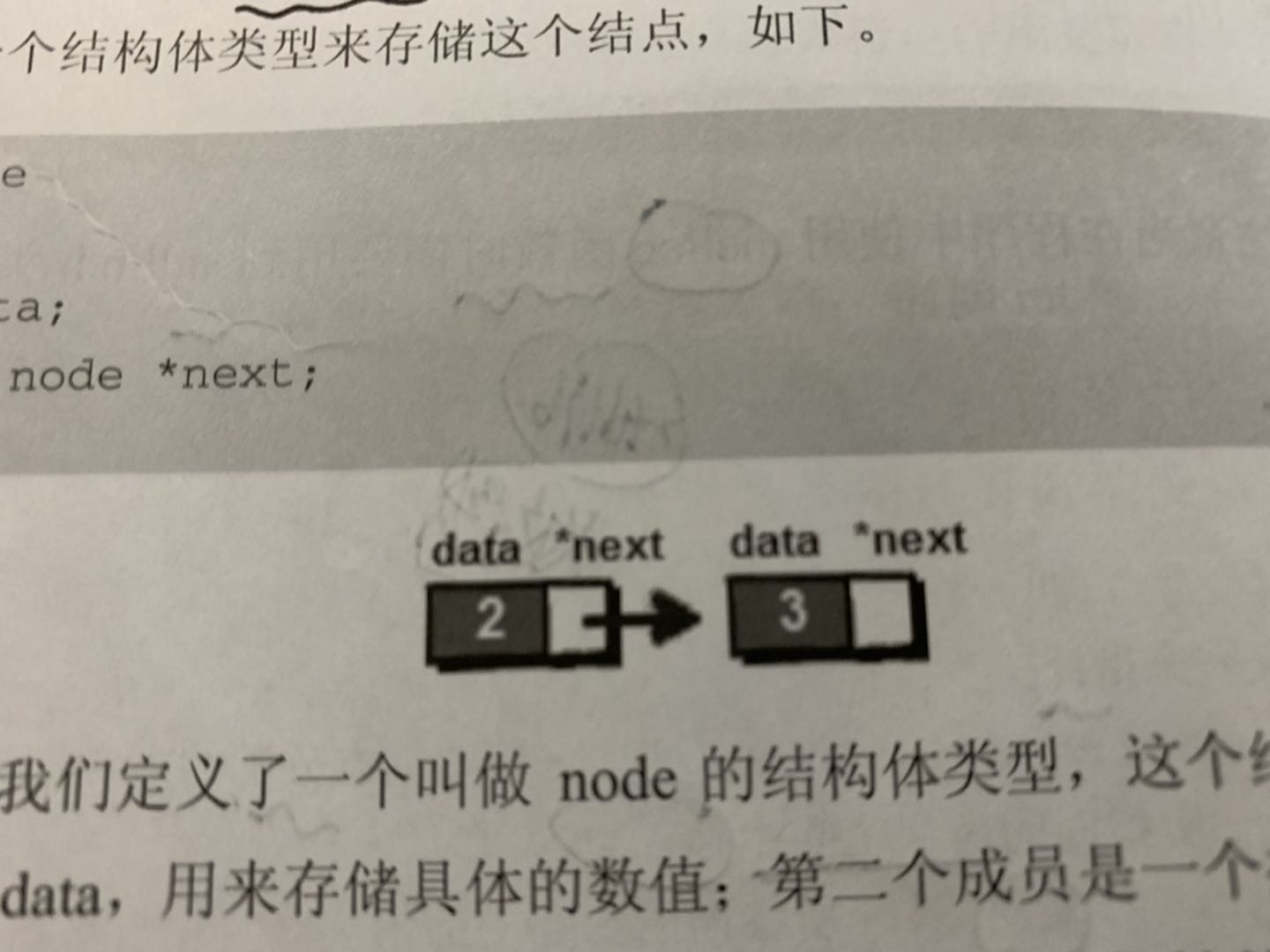# 指针

## 指针的定义

（点击代码框的text可以放大代码）

    int a;
int *p;
p=&a;     

    int  var1;
char var2;

printf("var1 变量的地址： %p\n", &var1  );
printf("var2 变量的地址： %p\n", &var2  );

return 0;

var1 变量的地址： 0x7fff5cc109d4
var2 变量的地址： 0x7fff5cc109de

## 指针的作用

1，以数组元素做实参，向形式参数传递的是数组的值
2、以数组名做实参，向形式参数传递的是数组的地址

        int balance = {1000, 2, 3, 17, 50};
avg = getAverage( balance, 5 ) ;
记住 ，数组名为该数组首元素的地址，访问到首个地址就可以访问所有数组的地址。
double getAverage(int *arr, int size)
{
int    i, sum = 0;
double avg;

for (i = 0; i < size; ++i)
{
sum += arr[i]; //注意 arr[i]和*(arr+i)等价  记住规则即可，本质是利用指针从头部开始遍历。
}
avg = (double)sum / size;

return avg;}

# 链表struct node
{
int data;
struct node *next;

}

那么现在让我们开始建立一个链表，首先，任何数据都要有一个开始访问的路，所以我们需要建立一个头指针。

struct node*p；
p=(struct node *)malloc(sizeof(struct node));//动态申请一个空间去存放节点，

    scanf("%d",&a);
p->data=a;
p->next=null;

if(head==null)

else
q->next=p //如果不是第一个结点，则把上一个结点的next指针指向当前结点，完成两个链表的拼接

#include<stdio.h>
#include<stdlib.h>
struct node
{
int data;
struct node *next;

} ;

int main()

int i,n,a;
scanf("%d",&n);
for(i=1;i<=n;i++)
{

scanf("%d",&a);
p=(struct node*)malloc(sizeof(struct node)); //
p->data=a;
p->next=NULL;

else
q->next=p;

q=p;

}

接下来，就是进行链表的遍历，这一块就比较方便了，只需要一个指针访问头指针然后从头开始遍历

}